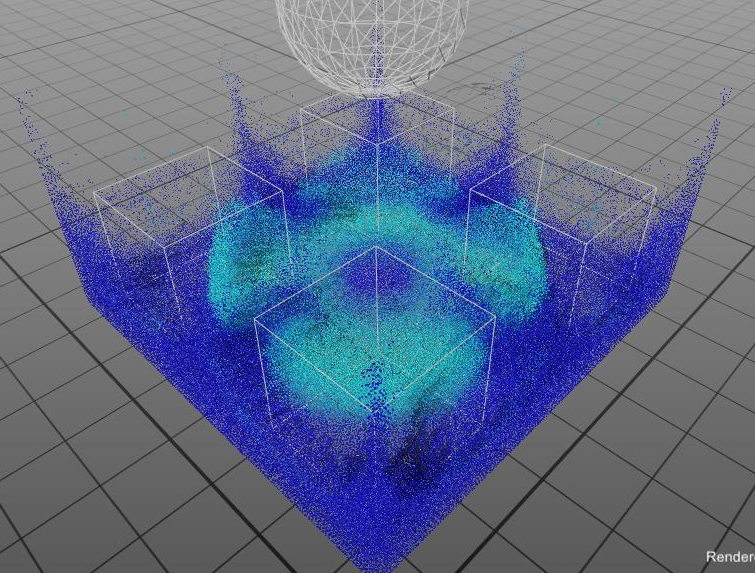# 基于位置约束的方法

#### 1. 简介#### 2. 方法

$${\rho_i = \sum_{j,r_{ij}<h}m_j \cdot W(r_{ij},h)}$$ (公式1)

$$C_i(\mathbf{x}+\triangle \mathbf{x}) = \frac{\rho_i(\mathbf{x +\triangle \mathbf{x}})}{\rho_0}-1=0$$ (公式3)

$${\triangle \mathbf{x_{i}}}= {\frac{1}{\rho_0}}\sum_{j,r_{ij}<h}(\lambda_j+\lambda_i)\nabla W(r_{ij}, h)，$$ $$\nabla W(r, h) = \frac{\mathrm{d} r }{\mathrm{d} \mathbf{x}} \frac{\mathrm{d} W(r ,h)}{\mathrm{d} r}$$ (公式4)

$$\lambda_i = - \frac{Ci(\mathbf{x})}{\sum_k|\nabla_k C_i|^2}$$ (公式5)

#### 3. 实现

PeriDyno中，PBF求解器的源文件为：

"Engine/Dynamics/Module/DensityPBD.cu";
"Engine/Dynamics/Module/DensityPBD.h";


PBF求解器的输入为不满足恒定密度约束的流体粒子的位置，输出为满足恒定密度约束的粒子位置，以及惯性作用下的粒子速度。##### a. 包含的模块：
• SummationDensity（密度计算模块）

  std::shared_ptr<SummationDensity<TDataType>> m_summation;


DensityPBD求解器使用该模块用于计算粒子的质量密度，并以此来计算恒定密度约束条件下粒子位置的修正值。

• SpikyKernel (核函数)

 SpikyKernel<Real> m_kernel;


该模块即为SPH法的核函数$W(r_{ij},h)$。

##### b. 变量：
• NeighborList (邻域粒子列表)

DEF_ARRAYLIST_IN(int, NeighborIds, DeviceType::GPU, "");


为了实现粒子各项物理量及其微分量的插值计算，计算过程需要使用到粒子的邻域列表。该邻域列表需要在每一帧计算开始时预先由邻域查找模块生成，邻域查找模块的位置为：

"Engine\Framework\Topology\NeighborPointQuery.h"
"Engine\Framework\Topology\NeighborPointQuery.cu"

• Velocity, Position (粒子速度与位置)

  DEF_ARRAY_IN(Coord, Velocity, DeviceType::GPU, "Input particle velocity");
DEF_ARRAY_IN(Coord, Position, DeviceType::GPU, "Input particle position");


Velocity为粒子的速度（$\mathbf{v}$）；Position为粒子空间位置 ($\mathbf{x}$)。

• 内部变量

      DArray<Real> m_lamda;
DArray<Coord> m_deltaPos;


m_lamda 为上述公式5中的 $\lambda$； m_deltaPos为公式4中的 $\triangle\mathbf{x}$；

##### c. 参数：
    int IterationNumber = 5;
Real RestDensity = 1000.0f;
Real SamplingDistance = 0.005f;
Real SmoothingLength = 0.01f;


• IterationNumber： 迭代次数（迭代次数越多，计算结果越精确，每一帧的密度误差越小）；
• RestDensity： 静止密度（即公式2中的$\rho_0$）；
• SamplingDistance： 粒子的初始间距；
• SmoothingLength： SPH法中的平滑距离/支持域半径，同时也是粒子的邻域半径。

##### d. 函数：
• K_ComputeLambdas： 该函数为公式5，用于计算 $\lambda$。
  template <typename Real, typename Coord>
__global__ void K_ComputeLambdas(...)

• K_ComputeDisplacement： 该函数为公式4，用于计算 $\triangle \mathbf{x}$。
  	template <typename Real, typename Coord>
__global__ void K_ComputeDisplacement(...)

• DP_UpdateVelocity： 该函数为用于计算惯性作用下粒子的速度，即：$\mathbf{v}^{new}_i=\mathbf{v}^{old}_i + \triangle \mathbf{x_i}/{\delta t}$。
  template <typename Real, typename Coord>
__global__ void DP_UpdateVelocity(...)


#### 参考文献

 Macklin M , M Müller. Position based fluids[J]. Acm Transactions on Graphics, 2013, 32(4):1-12.